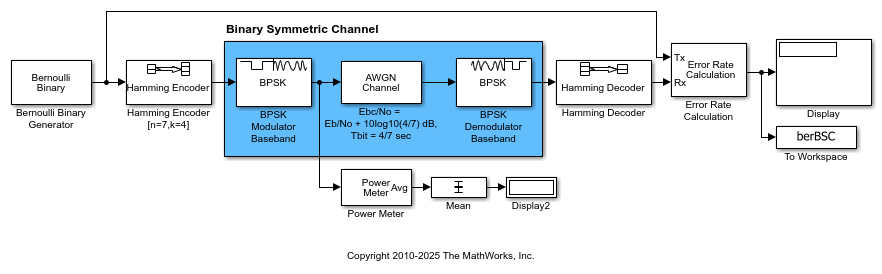Main Content

# Using AWGN Channel Block for Coded Signals

Two links perform error control coding on a signal that has passed through an impairment channel. Both links are set for an uncoded Eb/No of 8 dB.

In the top link, the AWGN channel block is set to provide a coded Eb/No of 8 + 10log10(4/7) dB, where 4/7 is the code rate. This accounts for the fact that the coded Eb/No is always lower (by a factor of the code rate) than the uncoded Eb/No. The blue shaded portion of the top link is simply a binary symmetric channel, which is modeled more compactly in the bottom link. The channel error probability of the top link is Q(sqrt(2*Ebc/No)), where Q() is the standard Q function and Ebc/No is the coded Eb/No (in absolute terms, not in dB).For this example, it is important to note that the bit period at the input of the AWGN Channel block is 4/7 sec. It is 1 sec at the input of the Hamming Encoder block, but that block decreases the bit time by a factor of the code rate.

If you allow the model to run for 1e6 bits, you'll note that the BERs are virtually identical. The difference lies in the stochastic nature of the two random number generators.

You can also check these BER results against expected analytical results by typing this command at the MATLAB® command prompt.

BER = bercoding(8,'block','hard',7,4,3)

This expression finds the upper bound of the BER of a linear, rate 4/7 block code with a minimum distance of 3, and hard decision decoding.

## Support

#### Bridging Wireless Communications Design and Testing with MATLAB

Download white paper